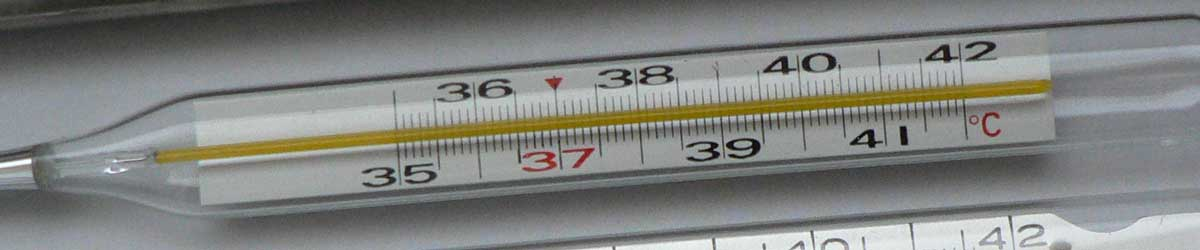# Newton to Delisle Conversion

Enter the temperature in Newton below to get the value converted to Delisle.

Results in Degrees Delisle:1 °N = 145.454545 °De
Do you want to convert Delisle to Newton?

## How to Convert Newton to DelisleConvert degrees Newton to degrees Delisle with this simple formula:

degrees Delisle = ( 33 - [°N] ) × 50 / 11

Insert the [°N] temperature measurement in the formula and then solve to find the result.

For example, let's convert 50 °N to degrees Delisle:

50 °N = ( ( 33 - 50 ) × 50 / 11 ) = -77.272727 °De

## Newton

The Newton scale is a temperature scale that sets a fixed zero point equal to the freezing point water. The scale uses both arithmetic progression and geometric progression. The scale defines 18 reference points and the corresponding "degrees of heat" and geometric progressions.

Newton can be abbreviated as N; for example, 1 degree Newton can be written as 1 °N.

## Delisle

The Delisle scale is a temperature scale that sets a fixed zero point equal to the temperature of boiling water. The Delisle scale is uses incrementing degrees as the temperature decreases. It runs from 0 degrees at the boiling point of water to 150 degrees at the the freezing point.

Delisle can be abbreviated as De; for example, 1 degree Delisle can be written as 1 °De.

### Important Temperatures In Degrees Delisle

TemperatureDegrees Delisle
Absolute Zero559.725 °De
Freezing Point of Water150 °De
Boiling Point of Water0 °De

## Newton to Delisle Conversion Table

Newton measurements converted to Delisle
Newton Delisle
-50 °N 377.27 °De
-40 °N 331.82 °De
-30 °N 286.36 °De
-20 °N 240.91 °De
-10 °N 195.45 °De
0 °N 150 °De
10 °N 104.55 °De
20 °N 59.09 °De
30 °N 13.64 °De
33 °N 0 °De
40 °N -31.82 °De
50 °N -77.27 °De
60 °N -122.73 °De
70 °N -168.18 °De
80 °N -213.64 °De
90 °N -259.09 °De
100 °N -304.55 °De
110 °N -350 °De
120 °N -395.45 °De
130 °N -440.91 °De
140 °N -486.36 °De
150 °N -531.82 °De
160 °N -577.27 °De
170 °N -622.73 °De
180 °N -668.18 °De
190 °N -713.64 °De
200 °N -759.09 °De
300 °N -1,214 °De
400 °N -1,668 °De
500 °N -2,123 °De
600 °N -2,577 °De
700 °N -3,032 °De
800 °N -3,486 °De
900 °N -3,941 °De
1,000 °N -4,395 °De